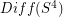# 4-sphere

## Is there an algorithm to determine if a triangulated 4-manifold is combinatorially equivalent to the 4-sphere? ★★★

Author(s): Novikov

Problem   Is there an algorithm which takes as input a triangulated 4-manifold, and determines whether or not this manifold is combinatorially equivalent to the 4-sphere?

Keywords: 4-sphere; algorithm

## What is the homotopy type of the group of diffeomorphisms of the 4-sphere? ★★★★

Author(s): Smale

Problemhas the homotopy-type of a product spacewhereis the group of diffeomorphisms of the 4-ball which restrict to the identity on the boundary. Determine some (any?) homotopy or homology groups of.

Keywords: 4-sphere; diffeomorphisms

## Which compact boundaryless 3-manifolds embed smoothly in the 4-sphere? ★★★

Author(s): Kirby

Problem   Determine a computable set of invariants that allow one to determine, given a compact boundaryless 3-manifold, whether or not it embeds smoothly in the 4-sphere. This should include a constructive procedure to find an embedding if the manifold is embeddable.

Keywords: 3-manifold; 4-sphere; embedding